Skip to main contentAn official website of the United States governmentOfficial websites use .gov
A .gov website belongs to an official government organization in the United States.Secure .gov websites use HTTPS
A lock ( ) or https:// means you’ve safely connected to the .gov website. Share sensitive information only on official, secure websites.

# NIST Demonstrates Key Step in Use of Quantum Computers for Code-Breaking

## ShareThis colorized image shows the fluorescence from three trapped beryllium ions illuminated with an ultraviolet laser beam. Black and blue areas indicate lower intensity, and red and white higher intensity. NIST physicists used three beryllium ions to demonstrate a crucial step in a procedure that could enable future quantum computers to break today's most commonly used encryption codes. Credit: NIST

Boulder, Colo.—A crucial step in a procedure that could enable future quantum computers to break today's most commonly used encryption codes has been demonstrated by physicists at the U.S. Commerce Department's National Institute of Standards and Technology (NIST).

As reported in the May 13 issue of the journal Science,* the NIST team showed that it is possible to identify repeating patterns in quantum information stored in ions (charged atoms). The NIST work used three ions as quantum bits (qubits) to represent 1s or 0s—or, under the unusual rules of quantum physics, both 1 and 0 at the same time. Scientists believe that much larger arrays of such ions could process data in a powerful quantum computer. Previous demonstrations of similar processes were performed with qubits made of molecules in a liquid, a system that cannot be expanded to large numbers of qubits.

"Our demonstration is important, because it helps pave the way toward building a large-scale quantum computer," says John Chiaverini, lead author of the paper. "Our approach also requires fewer steps and is more efficient than those demonstrated previously."

The NIST team used electromagnetically trapped beryllium ions as qubits to demonstrate a quantum version of the "Fourier transform" process, a widely used method for finding repeating patterns in data. The quantum version is the crucial final step in Shor's algorithm, a series of steps for finding the "prime factors" of large numbers—the prime numbers that when multiplied together produce a given number.

Developed by Peter Shor of Bell Labs in 1994, the factoring algorithm sparked burgeoning interest in quantum computing. Modern cryptography techniques, which rely on the fact that even the fastest supercomputers require very long times to factor large numbers, are used to encode everything from military communications to bank transactions. But a quantum computer using Shor's algorithm could factor a number several hundred digits long in a reasonably short time. This algorithm made code breaking the most important application for quantum computing.

Quantum computing, which harnesses the unusual behavior of quantum systems, offers the possibility of parallel processing on a grand scale. Unlike switches that are either fully on or fully off in today's computer chips, quantum bits can be on, off, or on and off at the same time. The availability of such "superpositions," in addition to other strange quantum properties, means that a quantum computer could solve certain problems in an exponentially shorter time than a conventional computer with the same number of bits. Researchers often point out that, for specific classes of problems, a quantum computer with 300 qubits has potentially more processing power than a classical computer containing as many bits as there are particles in the universe.

Harnessing all this potential for practical use is extremely difficult. One problem is that measuring a qubit causes its delicate quantum state to collapse, producing an output of an ordinary 1 or 0, without a record of what happened during the computation. Nevertheless, Shor's algorithm uses these properties to perform a useful task. It enables scientists to analyze the final quantum state after the computation to find repeating patterns in the original input, and to use this information to determine the prime factors of a number.

The work described in the Science paper demonstrated the pattern-finding step of Shor's algorithm. This demonstration involves fewer and simpler operations than those previously implemented, a significant benefit in designing practical quantum computers.

In the experiments, NIST researchers performed the same series of operations on a set of three beryllium qubits thousands of times. Each set of operations lasted less than 4 milliseconds, and consisted of using ultraviolet laser pulses to manipulate individual ions in sequence, based on measurements of the other ions. Each run produced an output consisting of measurements of each of the three ions. The NIST team has the capability to measure ions' quantum states precisely and use the results to manipulate other ions in a controlled way, before the delicate quantum information is lost.

The same NIST team has previously demonstrated all the basic components for a quantum computer using ions as qubits, arguably a leading candidate for a large-scale quantum processor. About a dozen different types of quantum systems are under investigation around the world for use in quantum processing, including the approach of using ions as qubits.

The new work was supported in part by the Advanced Research and Development Activity/National Security Agency.

As a non-regulatory agency, NIST develops and promotes measurement, standards and technology to enhance productivity, facilitate trade and improve the quality of life.

*J. Chiaverini, J. Britton, D. Leibfried, E. Knill, M.D. Barrett, R.B. Blakestad, W.M. Itano, J.D. Jost, C. Langer, R. Ozeri, T. Schaetz and D.J. Wineland. 2005. Implementation of the semiclassical quantum Fourier transform in a scalable system. Science. May 13, 2005.

Background
How the Quantum Fourier Transform Works

The classical Fourier transform, used in many fields of science and engineering, reveals patterns in a sequence of numbers. The quantum version of the Fourier transform reveals "periods," or repeating patterns, in quantum states. Such states might be prepared prior to the quantum Fourier transform, as in the NIST experiment reported in Science, or result from a calculation, such as one using Shor's algorithm.

Three classical bits can represent only one of eight possible values in binary notation (see box below, Counting with Qubits). Three qubits, in contrast, can represent up to eight values simultaneously, because they can be in superpositions of both 1 and 0 at the same time. Ions in a magnetic state called "spin up" are always 0s, and ions that are "spin down" are always 1s. Ions in a superposition state are both spin up and spin down at the same time. Scientists "measure" the state of a given ion by shining a laser on it, "collapsing" its quantum state to up or down. Ions in the spin down state emit light when illuminated with the right color of laser light, whereas ions in the spin up state do not.

Suppose the NIST experiment begins with two ions in superposition states (a combination of the "0" and "1" state simultaneously) and the third ion spin down (the "1" state). This means that when this set of ions is measured they will collapse to any one of four possible states. (See example graphic.)

The combined quantum state of the three ions in this example is the superposition of the states 001, 011, 101, and 111. This sequence corresponds to the classical numbers 1, 3, 5, and 7. After the NIST scientists manipulate the ions in the trap to perform the "Fourier transform"—which is essentially a mathematical computation—they get a result that reveals the length of this state's intrinsic repeating pattern. The scientists conducted the experiments with the ions in this starting position many times. At the end of the experiments they consistently found the first ion was spin down and the second two ions were spin up, the equivalent of the binary number 100, or the classical number 4. This result means that the quantum state includes a pattern that repeats four times within eight possible states (4/8). When the result is depicted graphically, it corresponds to a pattern that appears in regular periods of 2. (The "period" is always the inverse of the "frequency" determined in the first step.) (See graphic.)

Periods can be easy to see in small sets of numbers. But if the set of numbers is very large, like those used in encryption codes, methods such as the Fourier transform are essential. The quantum Fourier transform enables scientists to find periods in quantum states, a necessity for finding the factors of large numbers using Shor's algorithm.

 Example:At the beginning of the experiment, the first two ions are in superpositions, the third ion is spin down. There are four possible measurement outcomes.
 Counting with Qubits ↑= spin up↓= spin down Qubits BinaryCode Number ↑ ↑ ↑ = 000 = 0 ↑ ↑ ↓ = 001 = 1 ↑ ↓ ↑ = 010 = 2 ↑ ↓ ↓ = 011 = 3 ↓ ↑ ↑ = 100 = 4 ↓ ↑ ↓ = 101 = 5 ↓ ↓ ↑ = 110 = 6 ↓ ↓ ↓ = 111 = 7

Suppose the NIST experiment begins with two ions in superposition states (a combination of the "0" and "1" state simultaneously) and the third ion spin down (the "1" state). This means that when this set of ions is measured they will collapse to any one of four possible states. (See example graphic.)

The combined quantum state of the three ions in this example is the superposition of the states 001, 011, 101, and 111. This sequence corresponds to the classical numbers 1, 3, 5, and 7. After the NIST scientists manipulate the ions in the trap to perform the "Fourier transform"—which is essentially a mathematical computation—they get a result that reveals the length of this state's intrinsic repeating pattern. The scientists conducted the experiments with the ions in this starting position many times. At the end of the experiments they consistently found the first ion was spin down and the second two ions were spin up, the equivalent of the binary number 100, or the classical number 4. This result means that the quantum state includes a pattern that repeats four times within eight possible states (4/8). When the result is depicted graphically, it corresponds to a pattern that appears in regular periods of 2. (The "period" is always the inverse of the "frequency" determined in the first step.) (See graphic.)

Periods can be easy to see in small sets of numbers. But if the set of numbers is very large, like those used in encryption codes, methods such as the Fourier transform are essential. The quantum Fourier transform enables scientists to find periods in quantum states, a necessity for finding the factors of large numbers using Shor's algorithm.

 Example:At the beginning of the experiment, the first two ions are in superpositions, the third ion is spin down. There are four possible measurement outcomes.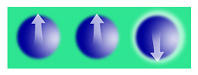= 001 = 1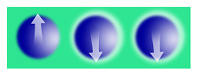= 011 = 3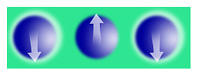= 101 = 5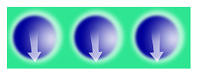= 111 = 7 Fourier operationsproduce this result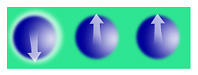= 100 = 4 Fourier transformreveals this pattern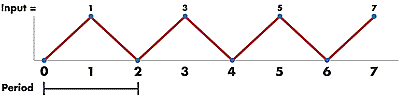Frequency = 4/8 Period = 2Repeating pattern occurs every 2 numbers
Released May 12, 2005, Updated January 8, 2018